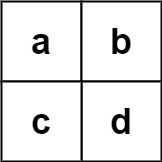# LC 212. 单词搜索 II

### 题目描述• m == board.length
• n == board[i].length
• 1 <= m, n <= 12
• board[i][j] 是一个小写英文字母
• 1 <= words.length <= 3 * $10^4$
• 1 <= words[i].length <= 10
• words[i] 由小写英文字母组成
• words 中的所有字符串互不相同

### 回溯算法

1. 如果当前爆搜到的字符串长度超过 $10$，直接剪枝；
2. 如果当前搜索到的字符串在 Set 中，则添加到答案（同时了防止下一次再搜索到该字符串，需要将该字符串从 Set 中移除）。

• 时间复杂度：共有 $m n$ 个起点，每次能往 $4$ 个方向搜索（不考虑重复搜索问题），且搜索的长度不会超过 $10$。整体复杂度为 $O(m n * 4^{10})$
• 空间复杂度：$O(\sum_{i=0}^{words.length - 1} words[i].length)$

### Trie

• 时间复杂度：共有 $m n$ 个起点，每次能往 $4$ 个方向搜索（不考虑重复搜索问题），且搜索的长度不会超过 $10$。整体复杂度为 $O(m n * 4^{10})$
• 空间复杂度：$O(\sum_{i=0}^{words.length - 1} words[i].length * C)$，$C$ 为字符集大小，固定为 $26$